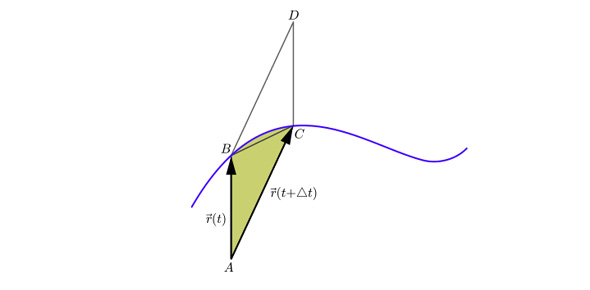# E1t7 Retake Preap 2016

Approved & Edited by ProProfs Editorial Team
The editorial team at ProProfs Quizzes consists of a select group of subject experts, trivia writers, and quiz masters who have authored over 10,000 quizzes taken by more than 100 million users. This team includes our in-house seasoned quiz moderators and subject matter experts. Our editorial experts, spread across the world, are rigorously trained using our comprehensive guidelines to ensure that you receive the highest quality quizzes.
| By Sfduke
S
Sfduke
Community Contributor
Quizzes Created: 57 | Total Attempts: 8,293
Questions: 5 | Attempts: 189Settings.

• 1.

### The jogger is at rest during which interval?

• A.

A. AB

• B.

B. BC

• C.

C. DE

• D.

D. EF

C. C. DE
Explanation
The jogger is at rest during the interval DE because the graph shows a horizontal line at the y-axis, indicating that the jogger is not moving. In intervals AB, BC, and EF, the graph shows an upward or downward slope, indicating that the jogger is in motion.

Rate this question:

• 2.

### During which of the following intervals does the jogger have a constant positive velocity?

• A.

A. BC

• B.

A. CD

• C.

C. DE

• D.

D. FG

A. A. BC
Explanation
In the given graph, the jogger has a constant positive velocity during the interval BC. This is because the graph shows a straight line with a positive slope during that interval, indicating that the jogger is moving at a constant speed in the positive direction.

Rate this question:

• 3.

### During which interval is the magnitude of the jogger’s acceleration the greatest?

• A.

A. AB

• B.

B. BC

• C.

C. DE

• D.

D. EF

D. D. EF
Explanation
The magnitude of the jogger's acceleration is the greatest during interval EF. This can be determined by looking at the graph provided. In interval EF, the graph shows a steep increase in the slope, indicating a rapid change in velocity. Since acceleration is the rate of change of velocity, a rapid change in velocity implies a large magnitude of acceleration. Therefore, interval EF has the greatest magnitude of acceleration.

Rate this question:

• 4.

### During which of the following intervals is the speed of the jogger decreasing?

• A.

A. AB only

• B.

B. AB and CD

• C.

C. AB, CD, and EF

• D.

D. EF only

B. B. AB and CD
Explanation
The speed of the jogger is decreasing during intervals AB and CD. This can be inferred from the given information that the jogger's speed is decreasing, which means the jogger is slowing down. Since the intervals AB and CD are the only intervals mentioned in the options where the jogger is not accelerating or maintaining a constant speed, it can be concluded that the speed is decreasing during these intervals.

Rate this question:

• 5.

### During which of the following intervals does the jogger have a constant negative velocity?

• A.

A. BC

• B.

B. CD

• C.

C. DE

• D.

D. FG

D. D. FG
Explanation
The jogger has a constant negative velocity during the interval FG because the position-time graph shows a straight line with a negative slope in that interval. This indicates that the jogger is moving in the negative direction at a constant speed.

Rate this question:

Related TopicsBack to top